# Scope

So what does this name refer to again?

## Definition

The scope of a binding is the region in a program in which the binding is active.

• Most languages employ static scoping (also called lexical scoping), meaning all scopes can be determined at compile time.
• Some languages (APL, Snobol, early LISP) employ dynamic scoping, meaning scopes depend on runtime execution.
• Perl lets you have both!

Regardless of whether scoping is static or dynamic, the definition implies that when you leave a scope, bindings are deactivated.

## Static Scope

Static scoping is determined by the structure of the source code:

```                   ┌──────────────────────────────────────────────┐
procedure P │ (X: Integer) is                              │
┌─────────────┘                                              │
│    A, B: Integer;                                          │
│                ┌────────────────────────────────────────┐  │
│    procedure Q │ (Y: Integer) is                        │  │
│  ┌─────────────┘ ┌───────────────────────────────────┐  │  │
│  │    function R │ (X, Y: Integer) return Integer is │  │  │
│  │  ┌────────────┘   ┌───────────────┐               │  │  │
│  │  │    procedure T │ is            │               │  │  │
│  │  │  ┌─────────────┘               │               │  │  │
│  │  │  │    T: Integer;              │               │  │  │
│  │  │  │ begin                       │               │  │  │
│  │  │  │    ...                      │               │  │  │
│  │  │  │ end T;                      │               │  │  │
│  │  │  └─────────────────────────────┘               │  │  │
│  │  │    W: Float;                                   │  │  │
│  │  │ begin                                          │  │  │
│  │  │    ...                                         │  │  │
│  │  │ end R;                                         │  │  │
│  │  └────────────────────────────────────────────────┘  │  │
│  │                ┌──────────────────────────────────┐  │  │
│  │    procedure S │ (Y: Integer) is                  │  │  │
│  │  ┌─────────────┘                                  │  │  │
│  │  │ begin                                          │  │  │
│  │  │   ...                                          │  │  │
│  │  │ end S;                                         │  │  │
│  │  └────────────────────────────────────────────────┘  │  │
│  │ begin                                                │  │
│  │    ...                                               │  │
│  │    declare X: Integer; begin ... end;                │  │
│  │    ...                                               │  │
│  │ end Q;                                               │  │
│  └──────────────────────────────────────────────────────┘  │
│    M: Integer;                                             │
│ begin                                                      │
│    ...                                                     │
│ end P;                                                     │
└────────────────────────────────────────────────────────────┘
```

The general rule is that bindings are determined by finding the “innermost” declaration, searching outward through the enclosing scopes if needed.

Interestingly, not everything is obvious! There are reasonable questions regarding:

• Where exactly the scope of a particular binding begins and ends
• Whether bindings that have been hidden in inner scopes are still accessible
• What actually counts as a declaration versus just a use? Can declarations be implicit?
• The effect of redeclarations within a scope

### Scope Range

Does the scope consist of a whole block or just from the declaration onward? Actually, there are at least four possibilities:

1. Scope is whole block (like JavaScript `let` or `const`), or the whole function (like JavaScript `var`)
2. Scope starts at beginning of declaration (like C)
3. Scope starts only after the whole declaration is finished (like Lua)
4. Scope starts after declaration is finished, but using occurrences of name are prohibited during declaration (like Ada)
Exercise: Consider this pseudocode:
```var x = 3
var y = 5
function f() {
print y
var x = x + 1
var y = 7
print x
}
f()
```
What is the effect of compiling and running the code under each of the four possibilities above?

### Scope Holes

In static scoping, “inner” declarations hide, or shadow outer declarations of the same identifier, causing a hole in the scope of the outer identifier’s binding. But when we’re in the scope of the inner binding, can we still see the outer binding? Sometimes, we can:

In C++:

```int x = 1;
namespace N {
int x = 2;
class C {
int x = 3;
void f() {int x = 4; cout << ::x << N::x << this->x << x << '\n';
}
}```

```procedure P is
X: Integer := 1;
procedure Q is
X: Integer := 2;
begin
Put(X);     -- writes 2
Put(P.X);   -- writes 1
end Q;
end P;```

However, some languages have restrictions on hiding declarations:

```void f() {
int x = 1;
if (true) {
int x = 2;
}
}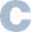```

is fine in C, but illegal in Java, because Java does not allow more than one declaration of a local variable within a method.

Exercise: Find out the rationale for this rule.

JavaScript has a somewhat interesting take on the interplay of scopes and nested blocks, with very different semantics between declarations introduced with `var`, `const`, or `let`.

Exercise: Research the difference between `var` and `let` in JavaScript, as it pertains to scope. You should run across something called the temporal dead zone (TDZ). Explain the TDZ in your own words.

### Indicating Declarations

Generally, languages indicate declarations with a keyword, such as `var`, `val`, `let`, `const`, `my`, `our`, or `def`. Or sometimes we see a type name, as in:

```int x = 5;              // C, Java```

or

```X: Integer := 3;        -- Ada```

When we use these explicit declarations in a local scope, things are pretty easy to figure out, as in this JavaScript example:

```let x = 1, y = 2;
function f() {
let x = 3;           // local declaration
console.log(x);      // uses local x
console.log(y);      // uses global y, it’s automatically visible
}
f();                   // writes 3 and 2```

Easy to understand, for sure, but be super careful, leaving off the declaration keyword in JavaScript updates, and introduces if necessary, a global variable:

```let x = 1;
function f() {
x = 2;                // DID YOU FORGET let?
}                       // Updated global var! Accident or intentional?
f()
console.log(x);```

Forgetting the declarating keywords has happened in the real world, with very sad consequences.

But in Python, there are no keywords and no types. Declarations are implicit. How does this work?

```x = -5
def f():
print abs(x)
f()                       # prints 5```

Sure enough we can see the globals `abs` and `x` inside `f`. Now consider:

```x = -5
def f():
x = 3
f()
print x                   # prints -5```

Aha, so if we assign to a variable inside a function, that variable is implicitly declared to be a local variable. Now how about:

```x = -5
def f():
print x
x = 3
f()
print x```

This gives `UnboundLocalError: local variable 'x' referenced before assignment`. The scope of the local `x` is the whole function body!

Exercise: Why do the Python rules “make sense”?
Exercise: Do some research for this one: How then do you write to a global variable from inside a function? Is there ever a good reason to do so?

Now Ruby’s declarations are also implicit. Let’s see what we can figure out here:

```x = 3
def f(); puts x; end      # Error!
f()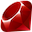```

WHOA! `NameError: undefined local variable or method `x' for main:Object`. We can’t see that outer `x` inside of `f`! If we want to play this game, we must use global variables, which in Ruby start with a `\$`:

```\$x = 3
def f(); x = 5; y = 4; p [\$x, x, y] end    # writes [3, 5, 4]
f()```

So assigning to a local declares it, and variables starting with a `\$` are global.

Exercise: Write a Ruby script to determine whether you can write to a global variable from within a function. Is there ever a good reason to do so?

CoffeeScript also has no explicit declarations. But it has a controversial design. If you assign to a variable inside a function, and that variable does not already have a binding in an outer scope, a new local variable is created. But if an outer binding does exist, you get an assignment to the outer variable.

```x = 3
f = () -> x = 5
f()                  # Plasters the global x
console.log(x)       # Writes 5

g = () -> y = 8
g()                  # Defines a local y which then goes away
console.log(y)       # Throws ReferenceError: y is not defined```

WTF? So if I write a little utility function that uses what I think are local variables, then I had better be damn sure that function does not find itself used in a context where it might actually affect global variables?

Exercise: Research this issue discussion on Github. Summarize the "problem" here. What major problems is Dmitry Soshnikov pointing out? Why does Jeremy Ashkenas think these are not real problems? Or are they?

### Redeclarations Within a Scope

If an identifier is redeclared within a scope, a language designer can choose to:

• Reject it (as a compile-time or run-time error).
• Make a fresh new binding and retain the old ones.
• Treat the redeclaration like an assignment.

Perl and ML actually retain the old bindings:

```# Perl
my \$x = 3;                # declare \$x and bind it
sub f {return \$x + 5;}    # uses the existing binding of \$x
my \$x = 2;                # declare a NEW entity and thus a new binding
print f(), "\n";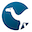```
```(* SML *)
val x = 3;                (* declare x and bind it *)
fun f() = x + 5;          (* uses existing binding of x *)
val x = 2;                (* declare a NEW entity and thus a new binding *)
f();```

Both of these programs print 8, because the bindings are new: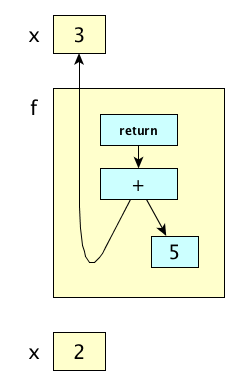But in some languages, the second declaration is really just an assignment, rather than the creation of a new binding. In such a language, the code implementing the above example would print 7, not 8.

Exercise: Find a language in which the direct translation of the code about would cause 7 to be printed. Write the program.

## Dynamic Scope

With dynamic scoping, the current binding for a name is the one most recently encountered during execution. The following script prints 2, not 1:

```# Perl
our \$x = 1;

sub f{
print "\$x\n";
}

sub g {
local \$x = 2;     # local makes dynamic scope (use my for static)
f();
}

g();
print "\$x\n";```

At each call, a new frame is created for each subroutine invocation and pushed on the call stack. A frame holds each of the locally declared variables for that invocation. In dynamic scoping, when looking up bindings for identifiers not in the current frame, we search the call stack. In our Perl example, while executing `f` and looking for `\$x`, we’ll find it in `g`’s frame: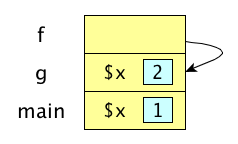Dynamic scopes tend to require lots of runtime work: type checking, binding resolution, argument checking, etc.

They are also prone to redeclaration problems.

Exercise: Explain this.

The principal argument in favor of dynamic scoping is the way they allow subroutines to be customized by using an “environment variable” style that allows selective overriding of a default (more or less). Michael Scott gives this example:

```# Perl
our \$print_base = 10;

sub print_integer {
...
# some code using \$print_base
...
}

# So generally we want to use 10, but say at one point
# we were in a hex mood.  We wrap the call in a block like this:
{
local \$print_base = 16;
print_integer(\$n);
}
# At the end of the block the old value is essentially restored```
Exercise: Rip this argument apart from the context of modern programming practice. Hints: Consider terms like “global variables” and “default parameters.”

## Implementing Scope Rules

In a statically scoped language, bindings are kept in a symbol table at compile time. You are free to throw away the names at runtime, unless you want to run the code under a good debugger. At run time, we can use static chains or displays. Details in the Compiler Construction course—next semester.

In a dynamically scoped language, you need to keep bindings at run time. The common approaches are to use (1) association lists or (2) a central reference table.

Here’s an example we’ll use to illustrate each approach.

```var i;
var j;
function p(var i) { ... }
function q(var j) {var x; ... p(j); ...}
q();
```

Let’s say main calls q, then q calls p.

### Association Lists

The association list, or A-list, works like this:

• When entering a scope: push its bindings on the list.
• When leaving a scope: pop its bindings off the list.
• When looking up a name: traverse the list.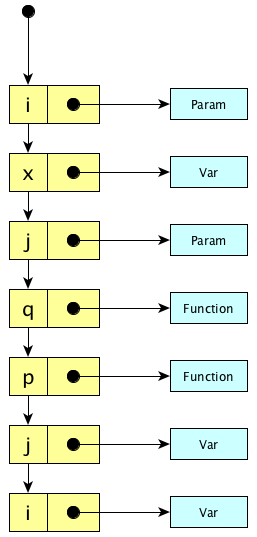Generally speaking, scope entry and exit is fast, lookup is slow.

Exercise: Really? What exactly is the entry/exit time proportional to? What exactly is the lookup time proportional to?

### Central Reference Table

The central reference table works like this:

• When entering a scope: push an entity descriptor onto each chain corresponding to each name defined in the scope.
• When leaving a scope: pop the relevant entity descriptors.
• When looking up a name: do a constant-time hashtable lookup. (It’s guaranteed to be Θ(1) because the correct entry is first on the list!)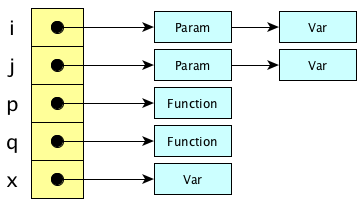Generally speaking, scope entry and exit is slow, lookup is blazingly fast (assuming your hash function is cheap).

Exercise: Really? What exactly is the entry/exit time proportional to?